# The city 2

Today lives 167000 citizens in city. How many citizens can we expect in 11 years if their annual increase is 1%?

Result

n =  186317

#### Solution:Leave us a comment of example and its solution (i.e. if it is still somewhat unclear...):

Showing 0 comments:Be the first to comment!#### To solve this example are needed these knowledge from mathematics:

Our percentage calculator will help you quickly calculate various typical tasks with percentages.

## Next similar examples:

1. The city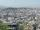At the end of 2010 the city had 248000 residents. The population increased by 2.5% each year. What is the population at the end of 2013?
2. The crime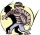The crime rate of a certain city is increasing by exactly 7% each year. If there were 600 crimes in the year 1990 and the crime rate remains constant each year, determine the approximate number of crimes in the year 2025.
3. Investment1000\$ is invested at 10% compound interest. What factor is the capital multiplied by each year? How much will be there after n=12 years?
4. Radioactive materialA radioactive material loses 10% of its mass each year. What proportion will be left there after n=6 years?
5. Machine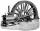Price of the new machine is € 62000. Every year is depreciated 15% of residual value. What will be the value of the machine after 3 years?
6. Enterprise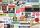The value of annual production enterprise is 7.1 million USD. The average annual growth rate is 1.5%. What will be value of production at the end of the fifth year?
7. Quotient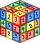Determine the quotient and the second member of the geometric progression where a3=10, a1+a2=-1,6 a1-a2=2,4.
8. Account operationsMy savings of php 90,000 in a bank earns 6% interest in a year. If i will deposit additional php 10,000 at the end of 6 months, how much money will be left if i withdraw php 25,000 after a year?
9. Geometric progression 2There is geometric sequence with a1=5.7 and quotient q=-2.5. Calculate a17.
10. Six termsFind the first six terms of the sequence a1 = -3, an = 2 * an-1
11. Profit gainIf 5% more is gained by selling an article for Rs. 350 than by selling it for Rs. 340, the cost of the article is:
12. Five membersWrite first 5 members geometric sequence and determine whether it is increasing or decreasing: a1 = 3 q = -2
13. Tenth memberCalculate the tenth member of geometric sequence when given: a1=1/2 and q=2
14. A perineumA perineum string is 10% shorter than its original string. The first string is 24, what is the 9th string or term?
15. Geometric sequence 4It is given geometric sequence a3 = 7 and a12 = 3. Calculate s23 (= sum of the first 23 members of the sequence).
16. Theorem proveWe want to prove the sentense: If the natural number n is divisible by six, then n is divisible by three. From what assumption we started?
17. GP - 8 itemsDetermine the first eight members of a geometric progression if a9=512, q=2